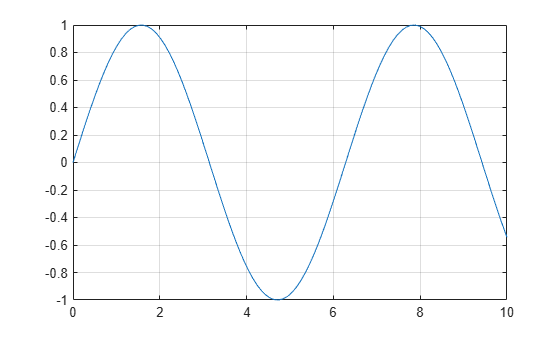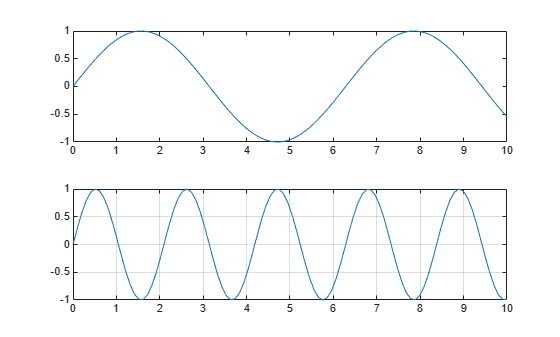# grid

Display or hide axes grid lines

## Syntax

``grid on``
``grid off``
``grid``
``grid minor``
``grid(target,___)``

## Description

example

````grid on` displays the major grid lines for the current axes returned by the `gca` command. Major grid lines extend from each tick mark.```

example

``grid off` removes all grid lines from the current axes or chart.`
````grid` toggles the visibility of the major grid lines.```

example

````grid minor` toggles the visibility of the minor grid lines. Minor grid lines lie between the tick marks. Not all types of charts support minor grid lines.```

example

````grid(target,___)` uses the axes or standalone visualization specified by `target` instead of the current axes. Specify `target` as the first input argument. Use single quotes around other input arguments, for example, `grid(target,'on')`.```

## Examples

collapse all

Display the grid lines for a sine plot.

```x = linspace(0,10); y = sin(x); plot(x,y) grid on```Create a surface plot and remove the grid lines.

```[X,Y,Z] = peaks; surf(X,Y,Z) grid off```Display the major and minor grid lines for a sine plot.

```x = linspace(0,10); y = sin(x); plot(x,y) grid on grid minor```Starting in R2019b, you can display a tiling of plots using the `tiledlayout` and `nexttile` functions. Call the `tiledlayout` function to create a 2-by-1 tiled chart layout. Call the `nexttile` function to create the axes objects `ax1` and `ax2`. Plot data into each axes. Then display grid lines in the bottom plot by passing `ax2` to the `grid` function.

```x = linspace(0,10); y1 = sin(x); y2 = sin(3*x); tiledlayout(2,1) % Top plot ax1 = nexttile; plot(ax1,x,y1) % Bottom plot ax2 = nexttile; plot(ax2,x,y2) grid(ax2,'on')```## Input Arguments

collapse all

Target object, specified as one of the following:

• Any type of axes object: an `Axes`, `PolarAxes`, or `GeographicAxes` object.

• A standalone visualization that has a `GridVisible` property. For example, a `heatmap` chart has a `GridVisible` property.

• An array of axes or standalone visualizations that belong to the same class. To determine the class, use the `class` function.

If you do not specify the target, then the `grid` function affects the graphics object returned by the `gca` command.

## Tips

• When working with geographic axes, some geographic axes properties affect the appearance of the grid lines. This table lists a subset of geographic axes properties related to the grid lines. Note that `GeographicAxes` objects do not support minor grid lines.

GeographicAxes PropertyDescription
`Grid`Display of latitude and longitude grid lines
`LineWidth`Line width of grid lines, box outline, and tick marks
`GridLineStyle`Grid line style
`GridColor`Color of grid lines
`GridAlpha`Grid line transparency

## Algorithms

The `grid` function sets these graphics object properties to either `'on'` or `'off'`:

### Topics

Introduced before R2006a